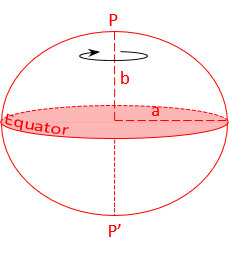### Article Index

This chapter is a simplified discussion of map projections. It covers systems typically used in surveying applications which are a small subset of the larger map making science. For those interested in more detail, "Map Projections - A Working Manual", USGS Prof Paper 1395 by John P. Snyder is an excellent technical resource. It thoroughly covers map projections and includes their equations with computational examples. At the time of this writing (11/2021) It was available as a free download at https://pubs.usgs.gov/pp/1395/report.pdf.

### 1.The Ellipsoid

The Earth is more complex than tomatoes or oranges and not a good mathematical model from which to project points. In the Horizontal Datums topic we saw how an ellipsoid, Figure D-1, was developed as a mathematical model of the Earth.Figure D-1 Ellipsoid
a, b are the semi-major and -minor axes, respectively

Heights, Figure D-2, allow us to project points from the physical earth to the ellipsoid.Figure D-2 Heights

Where

• H: orthometric height
• N: geoid height
• h: geodetic height

Once points are on the ellipsoid, they can be mathematically projected to a developable surface from which a 2D grid can be constructed.